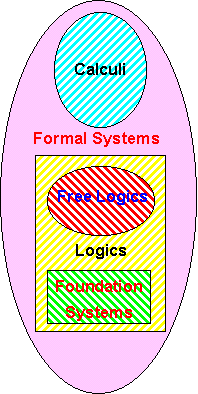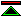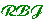# Formal Foundation Systems for MathematicsA formal system is a collection of sentences in some formal language together with a method of constructing demonstrations of some subset of the sentences. Demonstrable sentences are called theorems of the system and are normally intended to be some or all of the sentences which are true relative to some intended semantics for the sentences. Some, but not all of these systems are normally described as logics, others may be called calculi. The distinction is, however, rather loose and sometimes inconsistent (consider the lambda calculus and pure combinatory logic). Logics - Those formal systems normally described as logics fall into two main groups. Firstly there are simple systems such as propositional and modal logics, which, though limited in their expressiveness, are clearly concerned with reasoning rather than computation. Secondly there are the predicate logics, which are distinguished from calculi by containing constructs (such as quantifiers) which may not be computable, and therefore have the potential to demonstrate claims which cannot be established by brute computation. (e.g. that some algorithm does not terminate, or, Fermat's last theorem.). Since large parts of mathematics involve such claims, a logic proper, rather than a mere calculus, is needed for the formalisation of mathematics. Logics fall into three groups, free logics, foundation systems, and intermediate systems. Free Logics - A free logic embodies no presuppostions about what exists in the domain of discourse (the subject matter of sentences in the logic). Foundation Systems - Foundation Systems embody sufficiently lavish ontological presuppositions that a mathematician using them is unlikely to find further ontological premises necessary. A foundation system therefore provides a framework within which issues of consistency may be resolved by formal means. Calculi - The term calculus is used mainly for systems where the notation involved is essentially equivalent to a programming language, and the sentences demonstrated are typically equations asserting the claim that two expressions evaluate to the same value.©created 1995/12/29 modified 1996/3/30